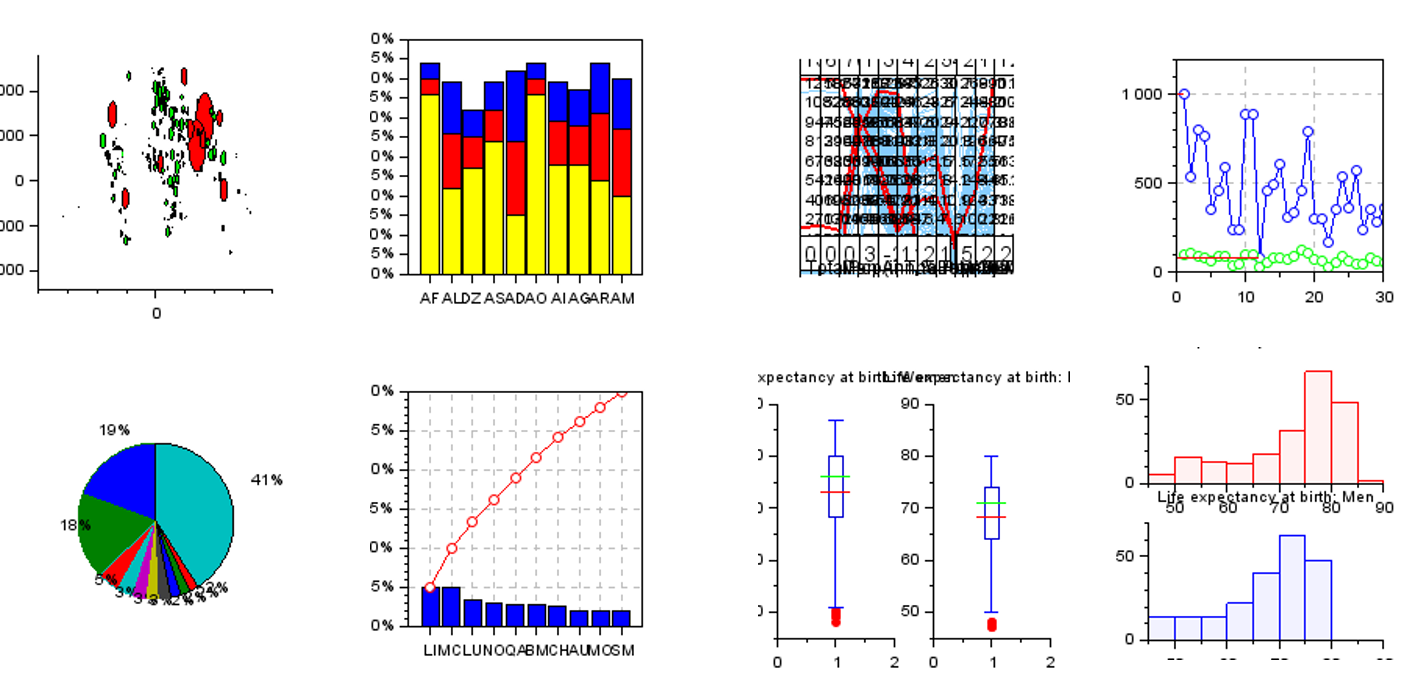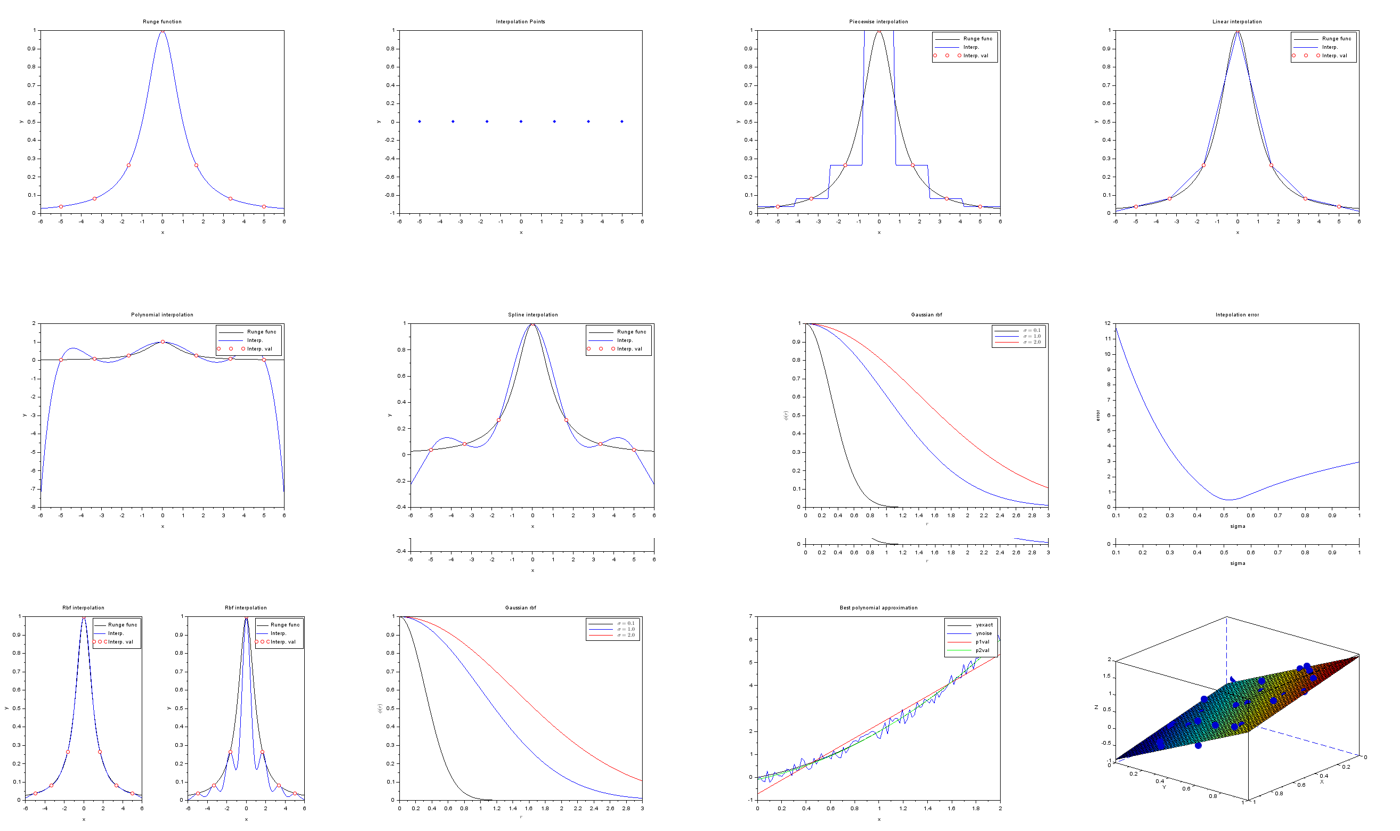# Statistics Training

## SCILAB training course for statistics

You might not be familiar with the numerical concept of statistics, but I am pretty sure your daily work requires you to:

• Evaluate and describe the numerics of your product or process
• Try to infer a model based on some measurements or analytical data## Training objective

Provide you with the basics to be able to:

1. Summarize your dataset through a few descriptive variables and infer a statistical model
2. Understand some basics on statistics theory and its numerical implementation
3. Qualify your problem to choose the most efficient method to solve it
4. Discover different methods implemented in Scilab and make efficient use of## Training content

 Basic data analysis Introduction to statistics problem in real life Data mining Data fitting Descriptive Statistics Numerical setting Introduction to statistics problem in mathematics & numeric Regression Classification Clustering The Design of Experiments Introduction to appropriate sampling of data from measurement or simulation (Factorial design, Box-Benhken, Central composite design, Latin Hypercube Sampling,...) Design techniques Experiment purpose Design purpose Meta-modeling & Validation Response Surfaces, Kriging, POD/SVD, Radial Basis Functions,...

## Training durationHugues-A. GARIOUD In charge of R&D at SCILAB Simulation, Optimization & Statistics email: